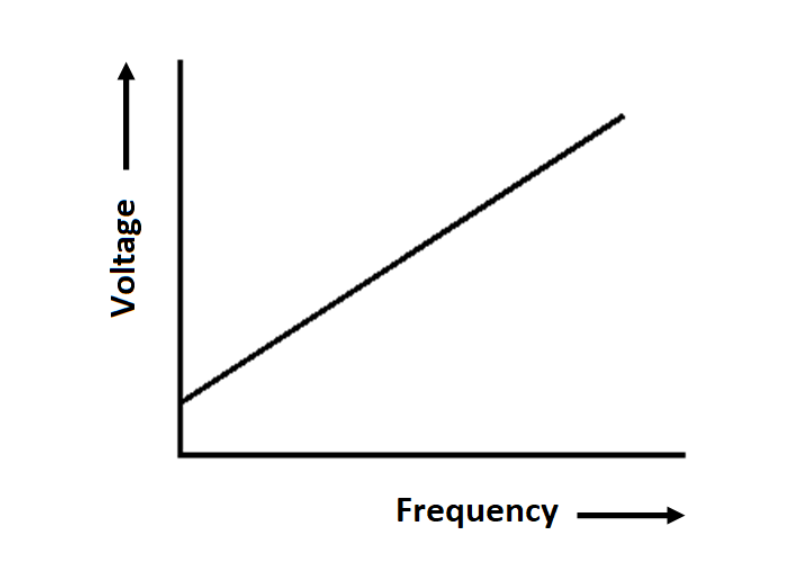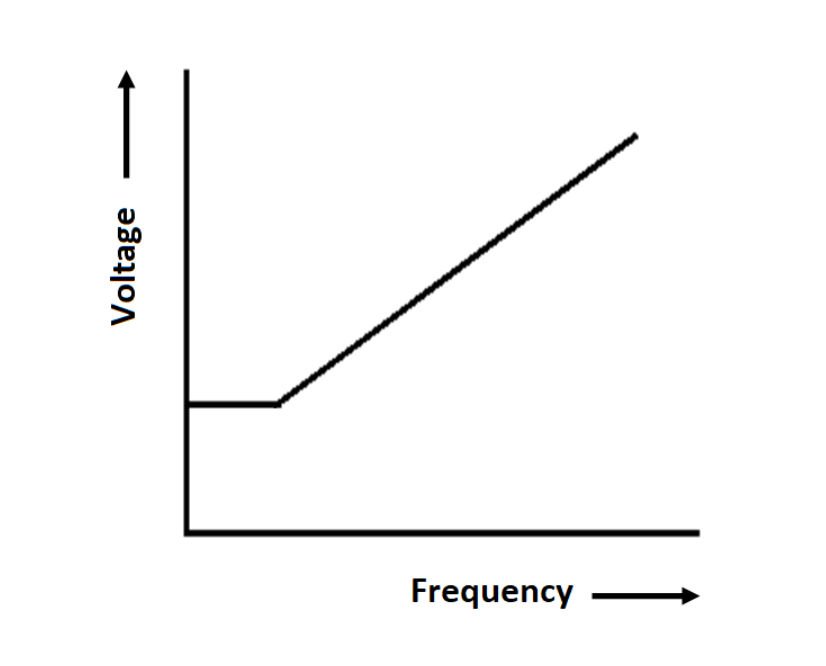# What is Torque Boosting in Variable Frequency Drive (VFD)?

## What is Motor Torque?

Variable frequency variable voltage (VVVF) or VFD is popularly used for driving the squirrel cage induction motor where speed control is desired to control the process. Substantial power saving can be achieved if the VFD is used for driving the centrifugal fans, blowers, and water pumps.

First, let us understand what the torque of an induction motor is and why starting torque is important for driving the mechanical equipment. When we feed the AC supply to the stator of the induction motor, magnetic flux is set up in the air gap length and the rotor voltage is induced.

The rotor of the motor is short-circuited at the end rings, and thus rotor current starts flowing in the rotor. The torque is produced in the motor because of the interaction of the magnetic flux and the rotor current.

The torque of induction current can be expressed by following mathematical expression.

T = K Φ Ir cos Φ equation (1)

The flux produced in the motor is proportional to the stator supply voltage.

Φ ∝ V

The rotor current is proportional to the slip and the stator supply voltage. At start slip=1,

Therefore,

Ir ∝ V

T = KV2 equation (2)

From the above equation, it is clear that the starting torque of the induction motor is proportional to the square of the stator voltage.

## How VFD Controls the Speed of Motor?

The voltage induced in the stator coil is equal to;

E = 4.44 Φ f T equation (3)

Φ = E / (4.44 f T)

Φ = V / (4.44 f T)

Φ = V / f equation (4)

The flux produced in the stator is proportional to the ratio of V/f. The speed of the motor can be expressed by following mathematical expressions.

Ns = (120 f) / P equation (5)

Thus the speed of the motor is proportional to the frequency.

V/f ratio is maintained constant to deliver the rated torque at all the speeds up to the base speed of the motor. If the V/f ratio is maintained constant, the motor operates in the constant torque mode.

## Why is High Starting Torque Required?

As per Newton’s laws of motion, the object which is in rest position tries to remain in its rest position if an external force is applied to it. This is called the law of inertia. The same principle applies when a motor drives a mechanical load.

The starting torque demand by the mechanical load depends on the combined moment of inertia of the motor and the driven equipment.

The more inertia, the more the starting torque required. If the motor does not supply the required starting torque then the motor will come to a stall.

## How VFD can Operate the Motor to supply High Starting Torque?

VFD generally operates in the constant torque mode by supplying constant volt/Hz to stator of the induction motor.The PWM inverter of the VFD increases the voltage and frequency in the same ratio, and thus the torque delivered by the motor remains constant.

For example, if the rated motor voltage and frequency of the motor are 400 volts and 50 Hz, then the V/f ratio is equal to 400/50=8. If the motor runs at half of its rated speed then the VFD output and frequency of the inverter are 200 volts and 50 Hz respectively and the V/f ratio is 200/25=8.

However, some types of loads demand high starting torque. To deliver the high starting torque, the v/f ratio of VFD output is deliberately made more than 8 for a definite time to generate more flux in the airgap.

More flux will lead to more torque than the motor-rated torque. V/f Pattern in the case of starting torque boosting is as given below.In the above V/f pattern, the voltage and frequency ratio is kept initially more to get more starting torque delivery.

Thus, the high inertial loads can be started by programming the V/f pattern in VFD. This feature of VFD is known as Torque Boosting Feature.

Source: <https://instrumentationtools.com/torque-boosting-in-variable-frequency-drive/>